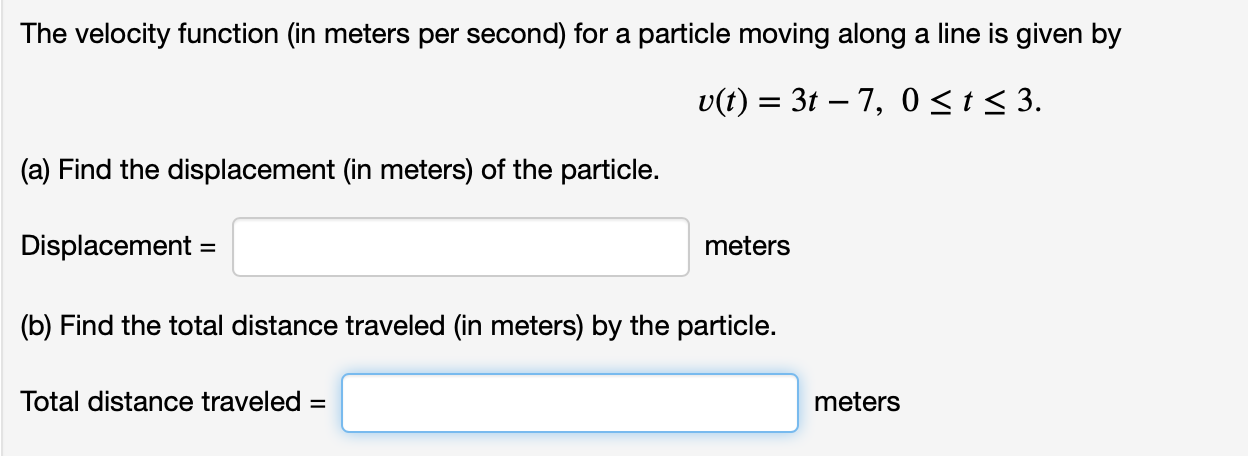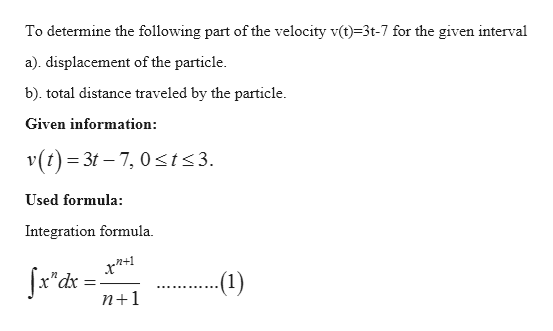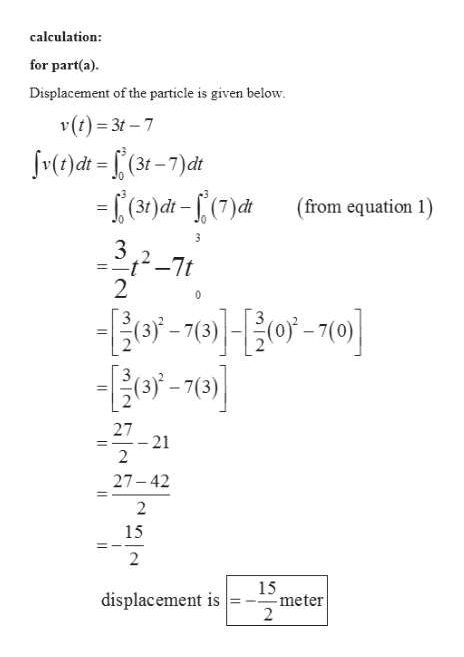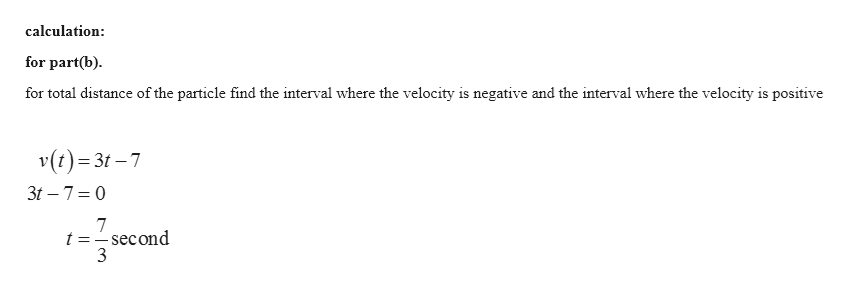# The velocity function (in meters per second) for a particle moving along a line is given byv(t) 3t 7, 0 < t < 3.(a) Find the displacement (in meters) of the particle.Displacement =meters(b) Find the total distance traveled (in meters) by the particle.Total distance traveled =meters

Question
16 viewshelp_outlineImage TranscriptioncloseThe velocity function (in meters per second) for a particle moving along a line is given by v(t) 3t 7, 0 < t < 3. (a) Find the displacement (in meters) of the particle. Displacement = meters (b) Find the total distance traveled (in meters) by the particle. Total distance traveled = meters fullscreen
check_circle

Step 1help_outlineImage TranscriptioncloseTo determine the following part of the velocity v(t)-3t-7 for the given interval a). displacement of the particle b). total distance traveled by the particle Given information v(t) 3t 7, 0 t<3. Used formula: Integration formula (1) n+1 fullscreen
Step 2help_outlineImage Transcriptionclosecalculation: for part(a) Displacement of the particle is given below (t)3t-7 v()dt=£(3-7)df (from equation 1) 3 - 7t 2 -70-70 27 = -21 2 27-42 2 15 2 15 meter 2 displacement is fullscreen
Step 3help_outlineImage Transcriptionclosecalculation for part(b) for total distance of the particle find the interval where the velocity is negative and the interval where the velocity is positive v(t) 3t7 3t 7 0 7 t =-second 3 fullscreen

### Want to see the full answer?

See Solution

#### Want to see this answer and more?

Solutions are written by subject experts who are available 24/7. Questions are typically answered within 1 hour.*

See Solution
*Response times may vary by subject and question.
Tagged in

### Calculus